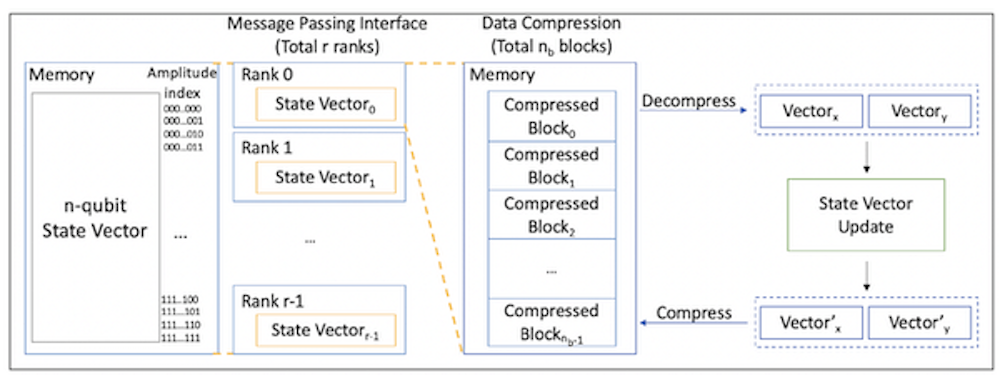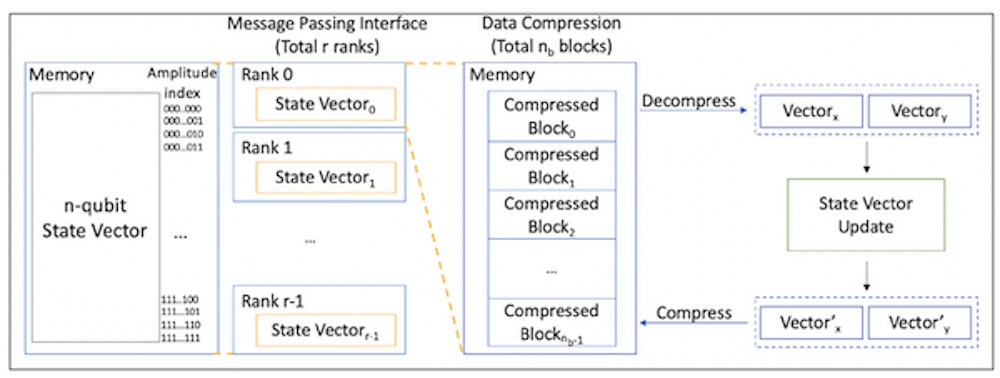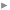Choose one...

# Researchers Simulate 61-Bit Quantum Computer with Data Compression

### When trying to debug quantum hardware and software by using a quantum simulator, every quantum bit (qubit) counts. Every simulated qubit closer to physical machine sizes halves the gap in compute power between the simulation and the physical hardware. However, the memory requirement of full-state simulation grows exponentially with the number of simulated qubits, and this limits the size of simulations that can be run.

Press release from Chicago Quantum Exchange
January 24th 2020 | 338 readersOverview of simulation with data compression. Image courtesy of Chicago Quantum Exchange.
Researchers at the University of Chicago and Argonne National Laboratory significantly reduced this gap by using data compression techniques to fit a 61-qubit simulation of Grover’s quantum search algorithm on a large supercomputer with 0.4 percent error. Other quantum algorithms were also simulated with substantially more qubits and quantum gates than previous efforts.

Classical simulation of quantum circuits is crucial for better understanding the operations and behaviors of quantum computation. However, today’s practical full-state simulation limit is 48 qubits, because the number of quantum state amplitudes required for the full simulation increases exponentially with the number of qubits, making physical memory the limiting factor. Given n qubits, scientists need 2^n amplitudes to describe the quantum system.

There are already several existing techniques that trade execution time for memory space. For different purposes, people choose different simulation techniques. This work provides one more option in the set of tools to scale quantum circuit simulation, applying lossless and lossy data compression techniques to the state vectors.

Figure 1 shows an overview of our simulation design. The Message Passing Interface (MPI) is used to execute the simulation in parallel. Assuming we simulate n-qubit systems and have r ranks in total, the state vector is divided equally on r ranks, and each partial state vector is divided into nb blocks on each rank. Each block is stored in a compressed format in the memory.

Read more from University of Chicago’s Department of Computer Science.

###Zapata AI, an Industrial Generative AI Software Company, to Go Public Through Business Combination with Andretti Acquisition Corp. - 09/09/2023

1 2 3 4 5 » ... 130

New comment: